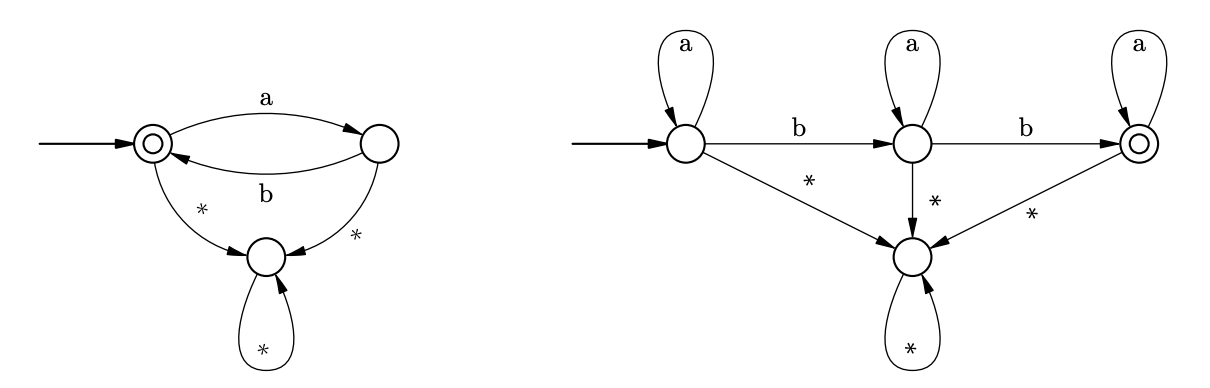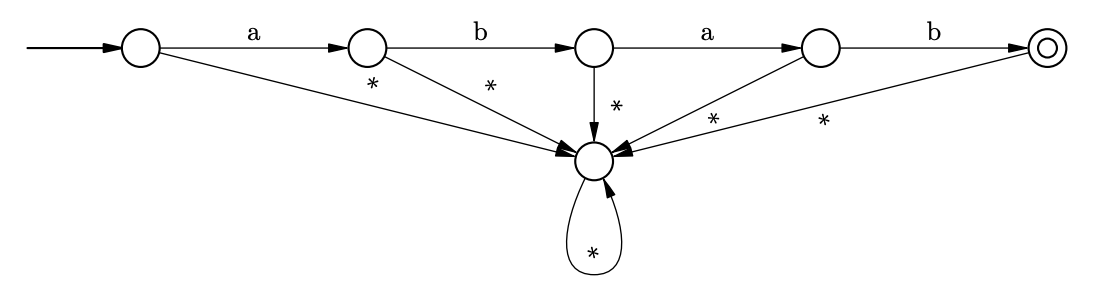시간 제한메모리 제한제출정답맞은 사람정답 비율
2 초 512 MB111100.000%

## 문제

Deterministic finite automaton (DFA) is an ordered set $\langle\Sigma, U, S, T, \varphi\rangle$ where $\Sigma$ is the finite set called input alphabet, in this problem $\Sigma = \{\mbox{a, b, ..., z}\}$, $U$ is the finite set of states, $S \in U$ is the initial state, $T \subset U$ is the set of terminal states and $\varphi : U \times \Sigma \rightarrow U$ is the transition function

The input of the automaton is the string $\alpha$ over $\Sigma$. Initially the automaton is in state $s$. Each step the automaton reads the first character $c$ of the input string and changes its state to $\varphi(u, c)$ where $u$ is the current state. Then the first character of the input string is removed and the step repeats. If after its input string is empty the automaton is in the terminal state, it accepts the initial string $\alpha$, in the other case it rejects it. The set of all words accepted by an automaton $A$ is denoted as $L(A)$.

One can visualize DFA as a directed graph representing its states as vertices and its transitions as edges marked with characters. Terminal states are shown as double circled vertices, the initial state is marked by an arrow. The picture below on the left shows the automaton for a language $(ab)^*$ of words that consist of zero or more repeated words "ab". The picture below on the right shows the automaton for a language $a^*ba^*ba^*$ that consist of "a" and "b" and contain two "b"-s. For sake of clarity edges marked by "$*$" represent all transitions not explicitly drawn.A set $X$ of words is called a regular language if it is equal to $L(A)$ for some DFA $A$. The index of a regular language $X$ denoted as $ind(X)$ is the minimal number of states in a DFA $A$ such that $L(A) = X$. For example, the two automatons shown on the picture above are indeed the minimal DFA-s for the described languages, so $ind((ab)^*)=3$ and $ind(a^*ba^*ba^*)=4$.

It is well known that if $X_1$ and $X_2$ are two regular languages its intersection $X_1 \cap X_2$ is also a regular language. For example, the intersection of the two languages described above is the language $Y=(ab)^*\cap\left(a^*ba^*ba^*\right)=\{abab\}$ that contains a single word "abab". Clearly a single word language is regular, the automaton for $Y$ is shown on the picture below.It is easy to see that if $W$ is a single word language $W = \{w\}$, and length of $w$ is $n$, the index of $W$ is equal to $n+2$.

A regular language $X$ is called decomposable if $X$ can be represented as an intersection of two regular languages $X = X_1 \cap X_2$ and $ind(X) > ind(X_1)$ and $ind(X) > ind(X_2)$. For example, the single word language $Y=\{\mbox{abab}\}$ is decomposable.

Given a word $w$ of length $n$ find whether the single word language $W = \{w\}$ is decomposable and if it is, find two automatons $A_1$ and $A_2$ such that number of states in both $A_1$ and $A_2$ is less than $n+2$ and $W = L(A_1)\cap L(A_2)$.

## 입력

The input file contains multiple test cases.

Each test case consists of a word $w$ on a line on itself, $w$ consists of lowercase letters of the English alphabet, length of $w$ is between 1 and 50, inclusive.

There are at most 100 tests in one input file.

## 출력

For each test case first print <<YES>> if the corresponding single-word language is decomposable, or <<NO>> if it is not. If the language is decomposable, the description of two DFA-s must follow. Each DFA description must start with $k$ --- the number of states, $1 \le k \le n + 1$, where $n$ is the length of the input word. Let states be numbered from 1 to $k$, the initial state is the state number 1. Then print $t$ --- the number of terminal states, $1 \le t \le k$, followed by $t$ integers from 1 to $k$ --- terminal states. The following $k$ lines must contain 26 integers each: for a state $u$ print $\varphi(u, \mbox{a})$, $\varphi(u, \mbox{b})$, $\dots$, $\varphi(u, \mbox{z})$.

## 예제 입력 1

abab
a


## 예제 출력 1

YES
3
1
1
2 3 3 3 3 3 3 3 3 3 3 3 3 3 3 3 3 3 3 3 3 3 3 3 3 3
3 1 3 3 3 3 3 3 3 3 3 3 3 3 3 3 3 3 3 3 3 3 3 3 3 3
3 3 3 3 3 3 3 3 3 3 3 3 3 3 3 3 3 3 3 3 3 3 3 3 3 3
4
1
3
2 1 4 4 4 4 4 4 4 4 4 4 4 4 4 4 4 4 4 4 4 4 4 4 4 4
3 2 4 4 4 4 4 4 4 4 4 4 4 4 4 4 4 4 4 4 4 4 4 4 4 4
4 3 4 4 4 4 4 4 4 4 4 4 4 4 4 4 4 4 4 4 4 4 4 4 4 4
4 4 4 4 4 4 4 4 4 4 4 4 4 4 4 4 4 4 4 4 4 4 4 4 4 4
NO